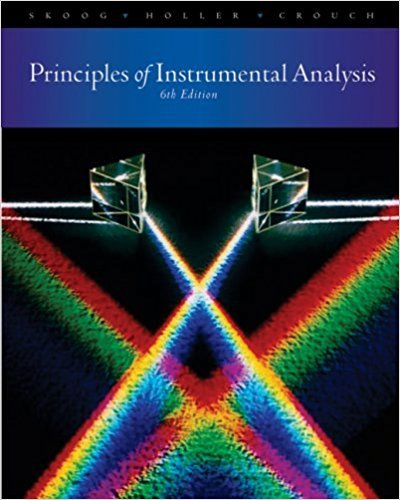×
×

# Solutions for Chapter 8: An Introduction to Optical Atomic .Spectrometry## Full solutions for Principles of Instrumental Analysis | 6th Edition

ISBN: 9780495012016Solutions for Chapter 8: An Introduction to Optical Atomic .Spectrometry

Solutions for Chapter 8
4 5 0 258 Reviews
28
0
##### ISBN: 9780495012016

Chapter 8: An Introduction to Optical Atomic .Spectrometry includes 12 full step-by-step solutions. Principles of Instrumental Analysis was written by and is associated to the ISBN: 9780495012016. Since 12 problems in chapter 8: An Introduction to Optical Atomic .Spectrometry have been answered, more than 13724 students have viewed full step-by-step solutions from this chapter. This expansive textbook survival guide covers the following chapters and their solutions. This textbook survival guide was created for the textbook: Principles of Instrumental Analysis , edition: 6.

Key Chemistry Terms and definitions covered in this textbook
• acid rain

Rainwater that has become excessively acidic because of absorption of pollutant oxides, notably SO3, produced by human activities. (Section 18.2)

• Aldehyde

A compound containing a !CHO group

• alpha (a) anomer

The cyclic hemiacetal of an aldose in which the hydroxyl group at the anomeric position is trans to the CH2OH

• bond order.

The difference between the numbers of electrons in bonding molecular orbitals and antibonding molecular orbitals, divided by two. (10.7)

• copolymer

A polymer that is constructed from more than one repeating unit.

• critical mass

The amount of fissionable material necessary to maintain a nuclear chain reaction. (Section 21.7)

• dienophile

A compound that reacts with a diene in a Diels-Alder reaction.

• diffusion.

The gradual mixing of molecules of one gas with the molecules of another by virtue of their kinetic properties. (5.7)

• hard water

Water that contains appreciable concentrations of Ca2 + and Mg 2 + ; these ions react with soaps to form an insoluble material. (Section 18.4)

• heat capacity

The quantity of heat required to raise the temperature of a sample of matter by 1 °C (or 1 K). (Section 5.5)

• Micelle

A spherical arrangement of organic molecules in water solution clustered so that their hydrophobic parts are buried inside the sphere and their hydrophilic parts are on the surface of the sphere and in contact with water

• node

Points in an atom at which the electron density is zero. For example, the node in a 2s orbital is a spherical surface. (Section 6.6)

• pi 1P2 bond

A covalent bond in which electron density is concentrated above and below the internuclear axis. (Section 9.6)

• probability density 1c22

A value that represents the probability that an electron will be found at a given point in space. Also called electron density. (Section 6.5)

• pyrometallurgy

A process in which heat converts a mineral in an ore from one chemical form to another and eventually to the free metal. (Section 23.2)

• Secondary (2°) amine

An amine in which nitrogen is bonded to two carbons and one hydrogen

• shielded

In NMR spectroscopy,protons or carbon atoms whose surrounding electron density is rich.

• SN1

A unimolecular nucleophilic substitution reaction.

• tertiary

A term used to indicate that exactly three alkyl groups are attached directly to a particular position. For example, a tertiary carbocation has three alkyl groups attached directly to the electrophilic carbon atom (C+).

• Wolff-Kishner reduction

Reduction of the C"O group of an aldehyde or ketone to a CH2 group using hydrazine and a base. Ylide (Section 16.6)

×